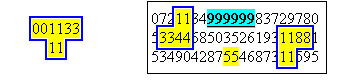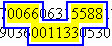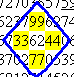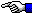# 1000 Decimal Places of Pi (with Color Highlighting)

 This table gives  p  to 1000 decimal places    nn = doubled-digits        nnn = tripled-digits p = 3. Decimal Places 14159265358979323846264338327950288419716939937510 58209749445923078164062862089986280348253421170679 82148086513282306647093844609550582231725359408128 48111745028410270193852110555964462294895493038196 44288109756659334461284756482337867831652712019091 45648566923460348610454326648213393607260249141273 72458700660631558817488152092096282925409171536436 78925903600113305305488204665213841469519415116094 33057270365759591953092186117381932611793105118548 07446237996274956735188575272489122793818301194912 98336733624406566430860213949463952247371907021798 60943702770539217176293176752384674818467669405132 00056812714526356082778577134275778960917363717872 14684409012249534301465495853710507922796892589235 42019956112129021960864034418159813629774771309960 51870721134999999837297804995105973173281609631859 50244594553469083026425223082533446850352619311881 71010003137838752886587533208381420617177669147303 59825349042875546873115956286388235378759375195778 18577805321712268066130019278766111959092164201989 50   100   150   200   250   300   350   400   450   500   550   600   650   700   750   800   850   900   950 1000

# Other Curiosities in the Digits of Pi ( Or: What's so Nifty about Rows of Fifty? )

When I created my first Pi-display some years ago with highlighted pairs of doubled-digits, I used 75 digits per line.  None of the highlighted pairs had to be split across lines, and I still like the way it appears.  If you look at the patterns of doubled-digits in that old display, there are a few that form what I like to think of as an upside-down perpendicular and two right angles:( These occur at the 360th through 365th decimal digits plus the 437th and 438th digits for the perpendicular shape.  Once you locate the string of six nines in a row, it's easy to find the two right angle shapes on either side of them. )

Are there any kind of shapes you can find in the display on this page (which uses 50 digits per line instead)?  Well, the 307th to the 310th and the 315th to the 318th decimal digits of Pi along with the 360th through the 365th digits form a sort of U-shaped pattern like this:If you look at the 459th and 460th decimal digits of Pi (which are 99 ), the 507th and 508th and 510th and 511th pairs (which are 33 and 44 ) and finally the 559th and 560th pair (which is 77 ), most likely you'll have already noticed that they form a diamond-shaped pattern like this:( Note: In the last 100 digits of Pi, I saw what may have been the beginning of another diamond, but the next line of 50 digits does not have a pair in the correct location to finish the pattern! )

If you'd like to search for other geometric patterns using the doubled-digits of Pi, here are some more HTML page displays to get you started:

60 digits per line
65 digits per line
70 digits per line
73 digits per line
75 digits per line
80 digits per lineThe Randomness of Pi (π)The Starman's Realm Index Page